# FRACTIONS Number Lines Whole Number on the Number

• Slides: 16FRACTIONS ØNumber Lines ØWhole Number on the Number Line ØIntegers on the Number Line ØFractions on the Number Line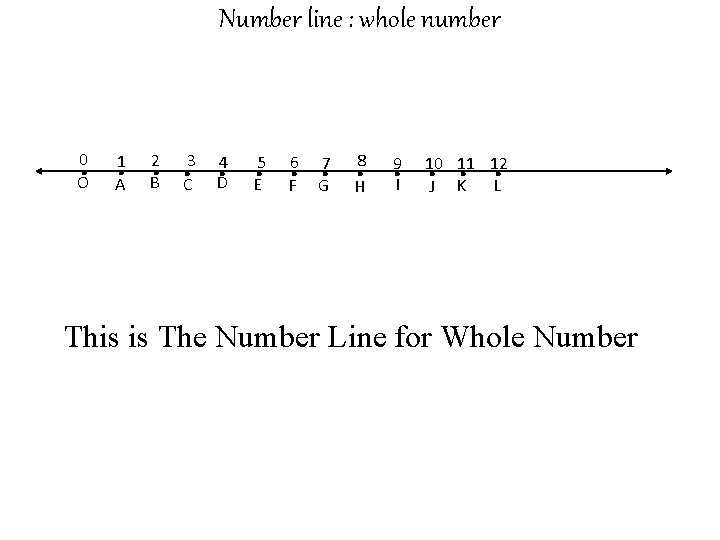Number line : whole number . . . 0 O 1 A 2 B 3 C 4 D 5 E 6 F 7 G 8 H 9 I 10 11 12 L J K This is The Number Line for Whole Number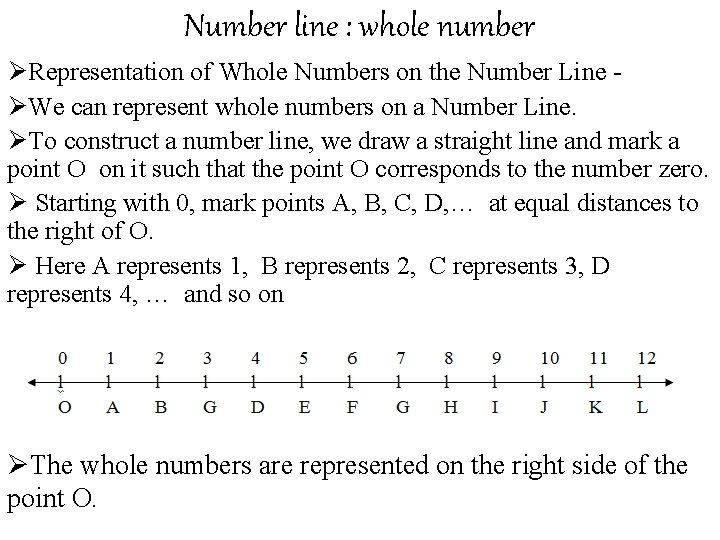Number line : whole number ØRepresentation of Whole Numbers on the Number Line ØWe can represent whole numbers on a Number Line. ØTo construct a number line, we draw a straight line and mark a point O on it such that the point O corresponds to the number zero. Ø Starting with 0, mark points A, B, C, D, … at equal distances to the right of O. Ø Here A represents 1, B represents 2, C represents 3, D represents 4, … and so on ØThe whole numbers are represented on the right side of the point O.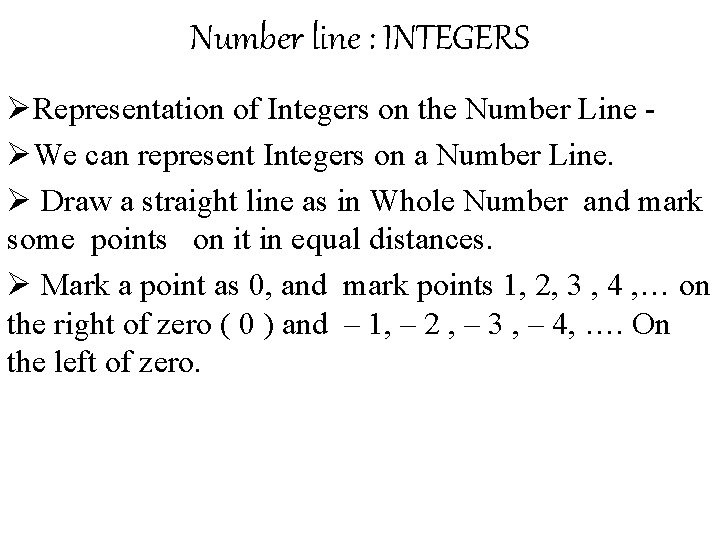Number line : INTEGERS ØRepresentation of Integers on the Number Line ØWe can represent Integers on a Number Line. Ø Draw a straight line as in Whole Number and mark some points on it in equal distances. Ø Mark a point as 0, and mark points 1, 2, 3 , 4 , … on the right of zero ( 0 ) and – 1, – 2 , – 3 , – 4, …. On the left of zero.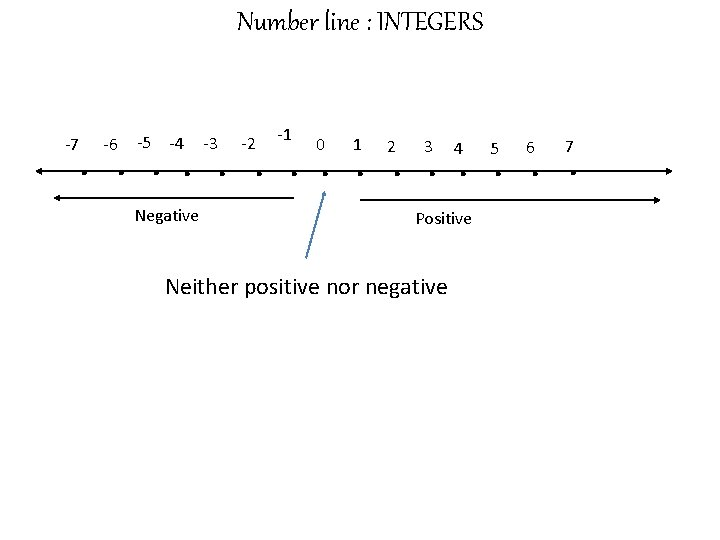Number line : INTEGERS -1 . . . . -7 -6 -5 -4 Negative -3 -2 0 1 2 3 4 Positive Neither positive nor negative 5 6 7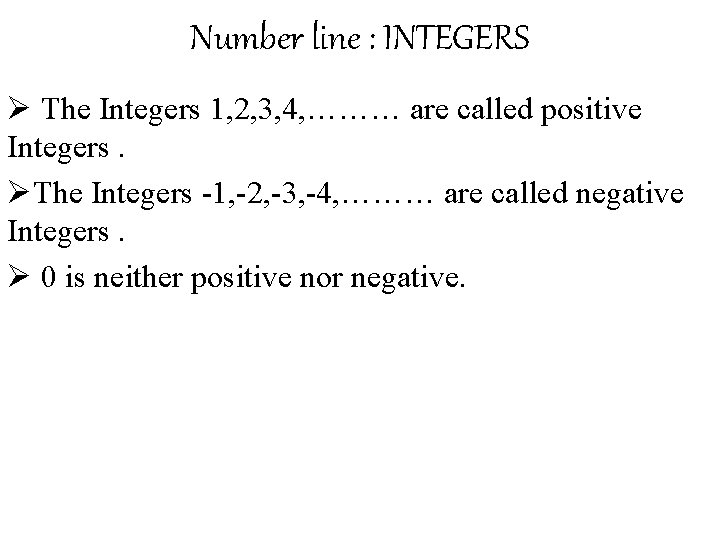Number line : INTEGERS Ø The Integers 1, 2, 3, 4, ……… are called positive Integers. ØThe Integers -1, -2, -3, -4, ……… are called negative Integers. Ø 0 is neither positive nor negative.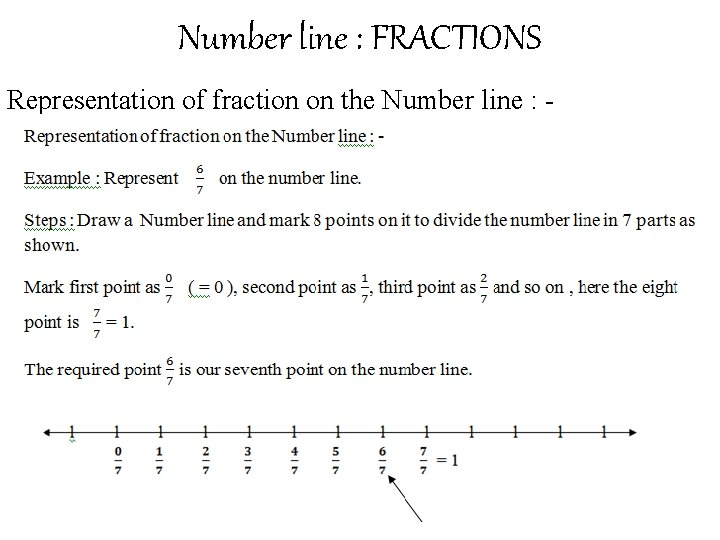Number line : FRACTIONS Representation of fraction on the Number line : -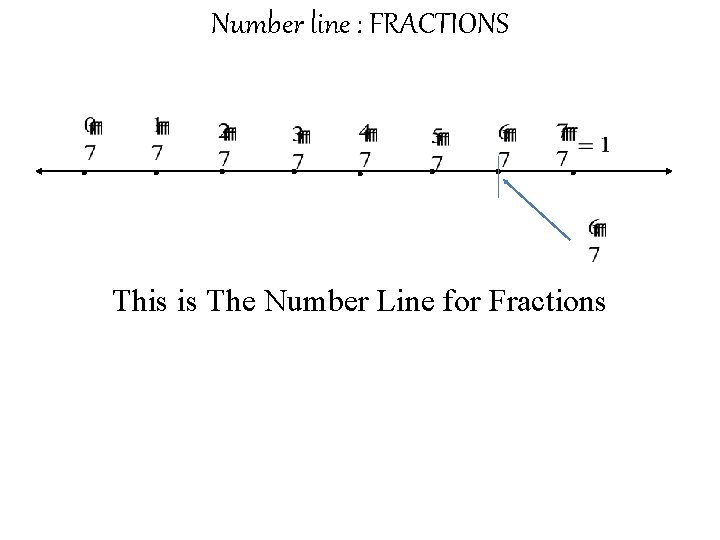Number line : FRACTIONS . . . . This is The Number Line for Fractions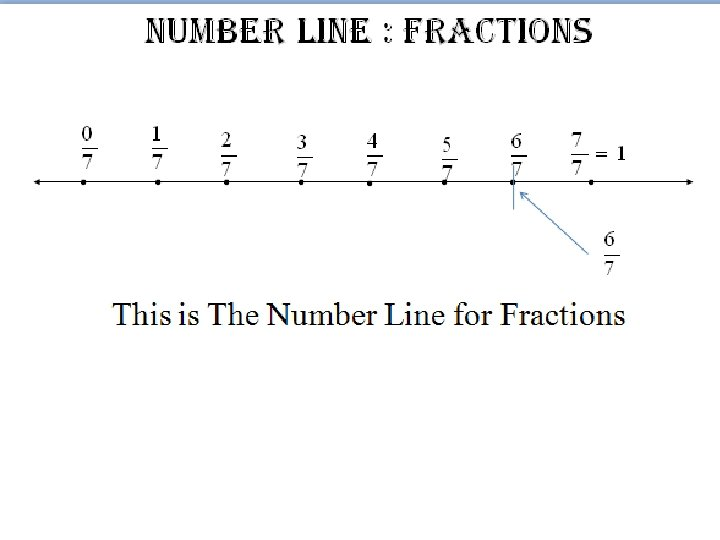Number line : FRACTIONS . . . . This is The Number Line for Fractions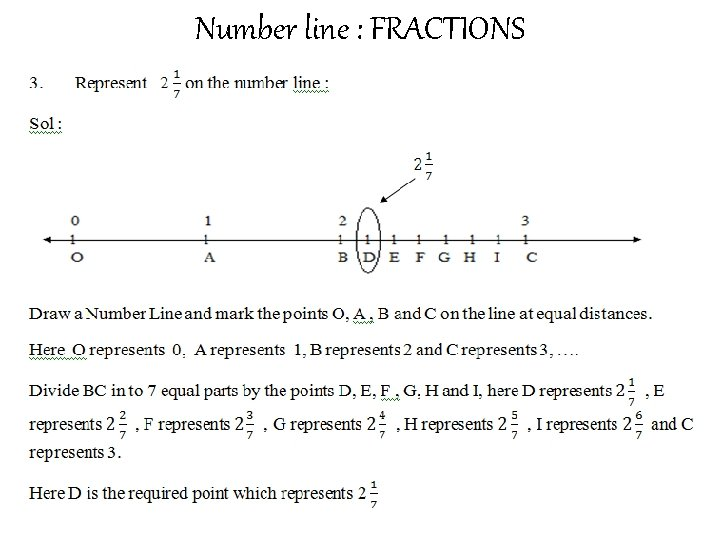Number line : FRACTIONSNumber line : FRACTIONS Represent . 0 O on the number line . A 1 . B. D E. F. G. H. . I C. 2 3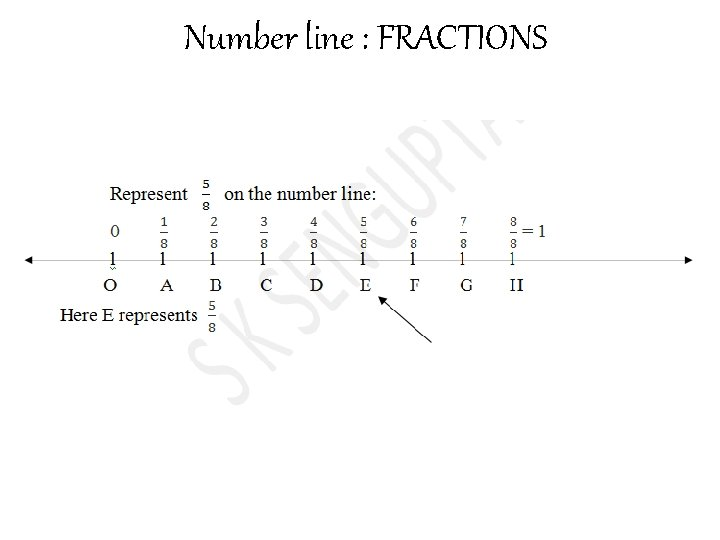Number line : FRACTIONS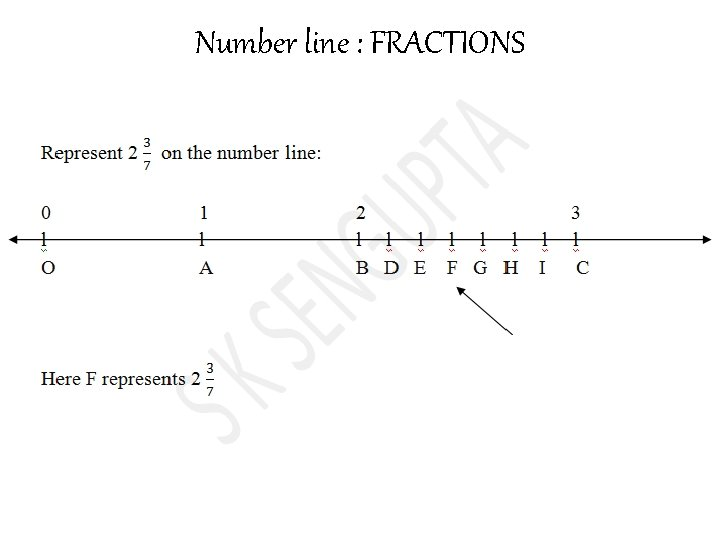Number line : FRACTIONS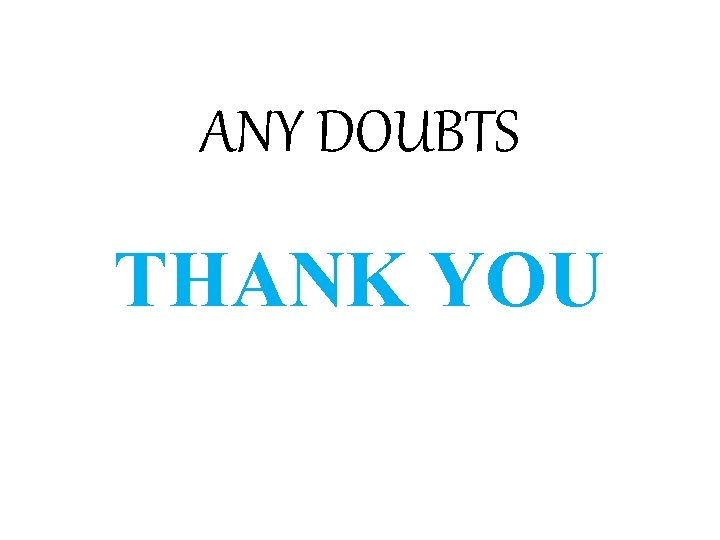ANY DOUBTS THANK YOU